# 7. Bouncing Around the Screen¶

In these notes you will learn:

• How to make a ball move in any direction at a constant speed.
• Some basic uses of if-statements.
• How to perform simple actions when edge is hit (such as stopping or expanding).

## 7.1. Introduction¶

Now lets create a program that makes a ball — or any shape or image — move in nay direction and bounce off any edge of the screen.

To allow a ball to move in any direction we need to take into account its speed in both the x-direction and the y-direction. Thus we will be using two variables, dx and dy, to control the ball’s speed. The variable dx will represent the change in the ball’s x-position, while the variable dy will represent the change in the ball’s y-position.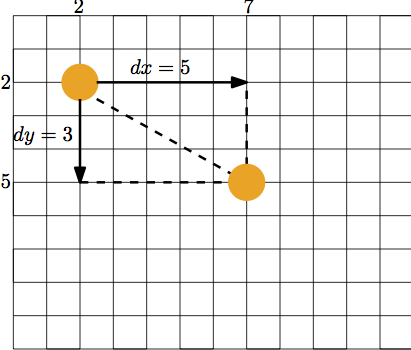In the above image, suppose that each square represents a pixel and that the ball starts at pixel (2, 2). Then the position of the ball the next time draw() is called will be (2 + dx, 2 + dy). With dx = 7 and dy = 3, the ball will be drawn in position (7, 5)

We’re also going to write the program so that every time the ball hits an edge it bounces off at an angle that is the same as the incoming angle. i.e.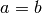in the following diagram: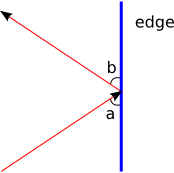Finally, we will ignore things like gravity and air friction. These details can be added later.

## 7.2. Variables and Initialization¶

Let’s walk thought the creation of our bouncing ball program step-by-step. First, we need a couple of variables to keep track of the position of the ball:

float x;
float y; //(x, y) is the center of the ball.


And then we need two speed variables to keep track of how far the ball along the x-axis and y-axis, on each call to draw():

float dx;
float dy;


For convenience, let’s also add a couple of colour variables:

color orange = color(255, 165, 0);
color light_yellow = color(255, 255, 165);


Note that all six of these variables are declared outsize of any function. That is they are all global variables.

Inside setup(), as usual, we initialize the screen and the fall ball variables:

void setup() {
size(500, 500);
smooth();

// set the ball's initial position and velocity
x = 250;
y = 250;

// move up and to the right
dx = 1;
dy = -2;
}


The precise starting values of x, ,y, dx, and dy, are, of course, entirely up to you.

## 7.3. Animating the Ball¶

We need to think about how to animate the ball. The essential idea is to repeat the following steps:

• draw the background
• draw the ball at (x, y)
• move the ball to its next position by adding dx to x and dy to y
• check if the ball has hit one of the four edges, and if so, change the direction of the ball by negating either dx or dy

The above way of describing a program is called pseudocode: it is a little bit like computer code, but written in English. We can also describe code using a flow chart. However, flow charts tend not to be used since they take up a lot more space than pseudocode without providing much (or any) more information.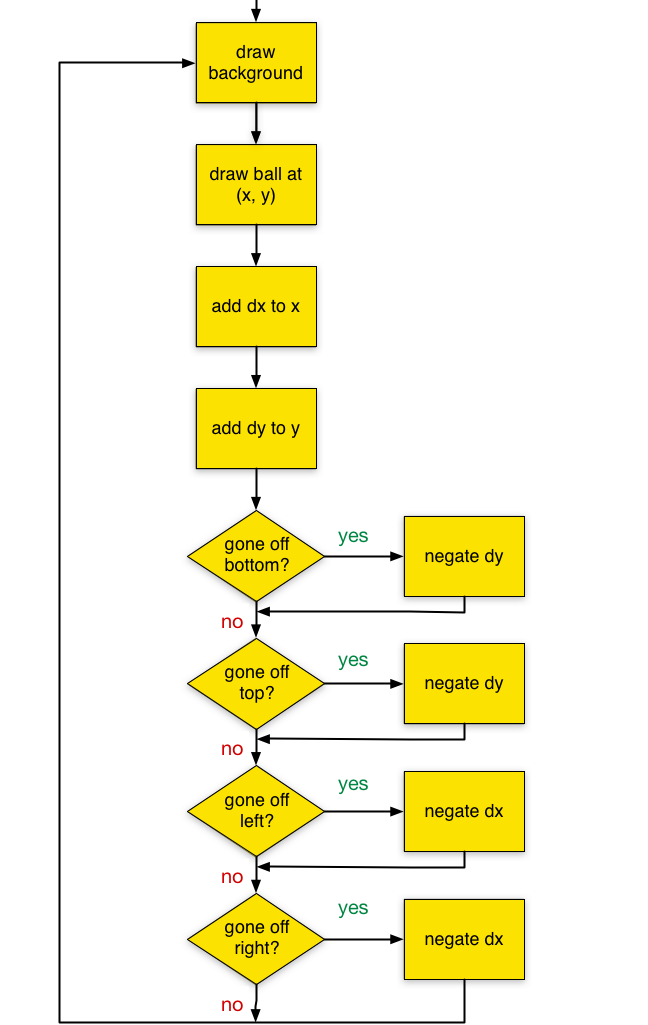Following the flow chart, the actual Processing code we write uses four if-statements, one for each edge:

void draw() {
// re-draw the background
background(light_yellow);

// draw the ball
noStroke();
fill(orange);
ellipse(x, y, 50, 50);

// move the ball to its next position.
x += dx;
y += dy;

// hit the top edge?
if (y - 25 <= 0) {
dy = -dy;
}

// hit the bottom edge?
if (y + 25 >= 499) {
dy = -dy;
}

// hit the left edge?
if (x - 25 <= 0) {
dx = -dx;
}

// hit the right edge?
if (x + 25 >= 499) {
dx = -dx;
}
}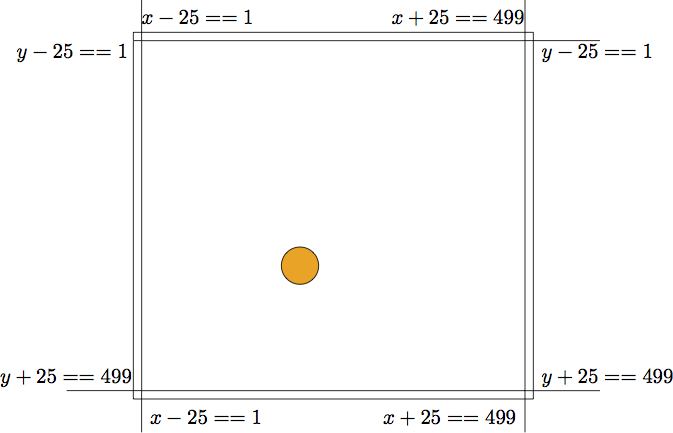## 7.4. Another Way to Write the If-statements¶

There’s often more than one correct way to write an if-statement. In our case, we can make use of the || operator to only write two separate if-statements:

if (y - 25 <= 0 || y + 25 >= 499) {
dy = -dy;
}

if (x - 25 <= 0 || x + 25 >= 499) {
dx = -dx;
}


Recall that the expression x - 25 <= 0 || x + 25 >= 499 is true either when x - 25 <= 0 is true, or when x + 25 >= 499 is true (or when both expressions are true). The only time it is false is when both x - 25 <= 0 is false and x + 25 >= 499 is false. i.e. x - 25 > 0 and x + 25 < 499. Thus we can simplify the code: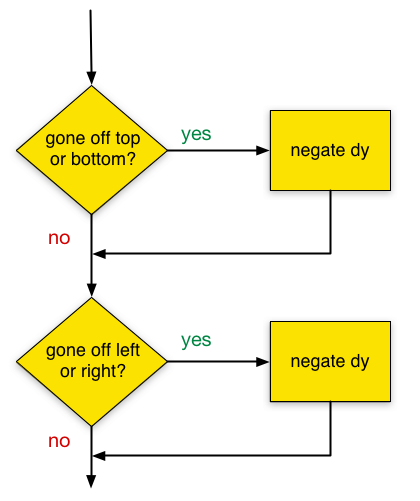It might seem that two if-statements are always preferable to four — it’s less code! But things aren’t that simple. First, the two if-statement version has more complicated conditions. More importantly though, what allowed us to use only two if-statements was the fact that what we wanted to do was symmetric: whether the ball hits the top or the bottom, its behaviour is altered in the same way. In general this will not necessarily be the case. Maybe the bottom edge is a pit, while the top edge is a ceiling, so that the ball should bounce off of the top edge, but fall off of the bottom edge. In this case you’ll need one if-statement for each kind of edge behaviour.

## 7.5. Stopping at the Top¶

Consider the next problem: how do we make the ball stop moving all together when it hits the top of the screen?

It turns out to be a simple change:

// hit the top edge?
if (y - 25 <= 0) {
dx = 0;
dy = 0;
}


We modify the code in the body of the if-statement that checks for hitting the top edge to set the speed of the ball to 0 in both directions. This causes the ball to stop as soon as it hits the top edge of the screen.

Keep in mind that even though the ball is not moving, draw() is still being called. The ellipse is still being drawn, and x and y are still being updated. But its position never changes because dx and dy are both 0.

## 7.6. Expanding When it Hits the Top¶

How can we make the ball bigger when it hits the top of the screen”? In other words, we want the diameter of the ball to get bigger every time it hits the top edge.

Currently, the diameter of the ball is fixed at 50:

ellipse(x, y, 50, 50);


Since our diameter is now going to change, we need to replace 50 with a variable that stores the current diameter. So let’s add the variable diam:

// ... variables as before ...

float diam; // Diameter of the ball

void setup() {
// .. code as before ..
diam = 50;
}

void draw() {
// ... code as before ...

// draw the ball
noStroke();
fill(orange);
ellipse(x, y, diam, diam);

// ... code as before ...
}


Now that the diameter of the ball is a variable, we can modify it when we need to:

// hit the top edge?
if (y = 25 <= 0) {
dy = -dy;
diam += 10;
}


Make this change and run the program, and you should see that the ball gets a little bit bigger each time it hits the top edge.

A problem you might notice is that part of the ball now goes off the edge of the screen. We didn’t have this problem when the diameter was fixed. What is going on?

The problem is cause by the if-statement conditions that check for edge collisions: they all assume that the diameter of the ball is fixed at 50. e.g.:

// hit the right edge?
if (x + 25 >= 499) {
dx = -dx;
}


The condition here is x + 25 >= 499, and we use x + 25 because that’s the x-coordinate of the right most point of the circle of diameter 50. But the diameter of our circle changes, depending on diam!. We must re-write the condition like this:

// hit the right edge?
if (x + diam / 2 >= 499) {
dx = -dx;
}


The expression x + diam / 2 is the x-value of the right most point of a ball of diameter diam: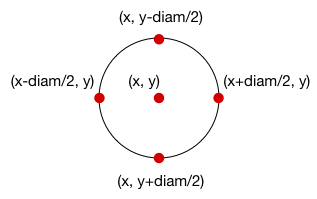So we need to adjust each if-statement to take diam into account:

// hit the top edge?
if (y - diam / 2 <= 0) {
dy = -dy;
diam += 10;
}

// hit the bottom edge?
if (y + diam / 2 >= 499) {
dy = -dy;
}

// hit the left edge?
if (x - diam / 2 <= 0) {
dx = -dx;
}

// hit the right edge?
if (x + diam / 2 >= 499) {
dx = -dx;
}


Does this work?

## 7.7. Debugging: Finding a Bug¶

Unfortunately, the above changes don’t quite work. When we run the modified program the ball gets stuck on top edge of the screen and inflates to a huge size without ever bouncing off any other edges. Obviously something is wrong with the program. But what?

This is a tricky error to deal with because there is no obvious flaw in any line of our code. The fact that the problem only occurs when the ball hits the top edge of the screen gives us a place to start:

// hit the top edge?
if (y - diam / 2 <= 0) {
dy = -dy;
diam += 10;
}


To figure out what is going on, it is helpful to know what lines of code are being called. A simple way to check this is to use a println statement to print a message every time the code in the if-statement body is called:

// hit the top edge?
if (y - diam / 2 <= 0) {
println("Hit the the top edge!");
dy = -dy;
diam += 10;
}


println prints a message in the black window at the bottom of the Processing editor (this window is known as the console window. We usually use println to help us understand and fix programs.

When you run the code after inserting this println, the ball still gets stuck and inflates at the top of the screen. But you should notice the following in the black window at the bottom:

hit top edge?
hit top edge?
hit top edge?
hit top edge?
...


What this tells us is that the body of the top-edge if-statement is being called again and again. Somehow, the top point of the ball has gotten stuck above the top edge of the screen and can’t get out.

How might this happen? Let’s try one more little trick: we’ll print the values of y, dy, and diam once, the first time the top-edge if-statement body gets executed, and then immediately stop the program:

if (y - diam / 2 <= 0) {
println("hit the top edge!");
println("diam = " + diam);
println("diam / 2 = " + diam / 2);
println("y = " + y);
println("dy = " + dy);
System.exit(0); // immediately stops the program

dy = -dy;
diam += 10;
}


Here’s the output that is produced on the console when the program runs:

hit top edge!
diam = 50.0
diam / 2 = 25.0
y = 24.0
dy = -2.0


This tells us the value that all the relevant variables are storing the first time that y - diam / 2 <= 0 evaluates to true. i.e. the first time the ball hits the top edge. The expression y - diam / 2 is less than 0 because y is 24 and diam / 2 is 25.

So far things are going as planned. Now let’s consider what happens next, imagining that we didn’t make the program exit using the call to System.exit(0). Then the next two statements are run:

dy = -dy;
diam += 10;


After these two lines execute, dy is set to 2, and diam is set to 60. But y is still 24. So what happens the next time draw() is called? We see that y will become 26 (since dy is 2), and when we get to the top-edge if-statement, we have that y - diam / 2 is equal to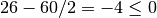, so that the condition of the if-statements is true again. Therefore, diam becomes 70 and dy becomes -2 (we ignore the extra lines we added for debugging). But then the next time draw() is called, y will go back to 22, and the condition y - diam / 2 <= 0 will be true again.

Thus we have a situation in which y oscillates between 22 and 24. Further, since diam increases with each call, the expression y - diam / 2 <= 0 evaluates to true at each call.

This is a tricky problem to catch. To understand it, you need to trace through a few calls to draw() to see that the values of y are stuck at 24 and 26. These sorts of errors get easier to catch (and avoid) with experience, but but you can never truly get rid of them: learning strategies for finding and fixing subtle bugs is an important part of programming.

“If debugging is the process of removing bugs, then programming must be the process of putting them in.”

Edsger W. Dijkstra

## 7.8. Debugging: Fixing the Problem¶

So we’ve identified the problem, but how do we fix it? This is not completely obvious because there are many ways that we could modify the value of the y (or even dy) variable that might fix it.

Let’s try to understand a little more of what is going on. The first time that the top-edge if-statement condition is true, the image on the screen looks something like this: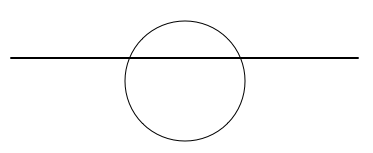That is, despite our arithmetic, the ball still goes a little bit into the top edge of screen (why does our arithmetic fail?). This is known as interpenetration. Since we are thinking of the ball and the edge as two solid objects, no interpenetration should be allowed.

The solution we’ll use is as follows: when the ball interpenetrates an edge, we’ll reset its position so that it just barley touches the edge. For example:

// hit the left edge?
if (y - diam / 2 <= 0) {
dy = -dy;
diam += 10;
y = diam / 2;
}


In this if-statement, when the ball hits the left edge of the screen we revers its direction, and then set x so that the ball is just touching the edge without ever going over. We are adjusting the position of the ball every time it hits an edge.

This fixes the problem in our case. However, we still may have interpenetration that occurs at the other edges. e.g. at the bottom edge, or should we change dx, at the left and right edges. So to be on the safe side, we’ll modify all of the edge related if-statements:

// hit the bottom edge?
if (y + diam / 2 >= 499) {
dy = -dy;
y = 499 - diam / 2 // Note the difference
}

// hit the left edge?
if (x - diam / 2 <= 0) {
dx = -dx;
x = diam / 2;
}

// hit the right edge?
if (x + diam / 2 >= 499) {
dx = -dx;
x = 499 - diam / 2;
}


## 7.9. Questions¶

1. What is pseudocode?

2. Pseudocode and flow charts can both be used to represent programs. List the pros and cons of each.

3. For each of the following expressions, state if it is true or false. Assume that x, y, dx, and dy are defined as follows:

float x = 32;
float y = -4;
float dx = 1.22;
float dy = 1.57;

1. x == x
2. x == y
3. dx < dy
4. (dy > dx) || (dy < dy)
5. (y == -4) || (x > 0)
6. (y < -4) || (x <= 0)
7. (x == y) || (dx == dy) || (x + y == dx + dy)
8. (1 <= 2) || (x > x)
9. (x < 0) || (x > 499) || (y < 0) || (y > 499)
10. (x > mouseX) || (y > mouseY)
4. Draw a flow chart for the following code snippet:

if (y <= 0) {
dy = -dy;
} else if (y >= 499) {
dy = -dy;
} else if (x <= 0) {
dx = -dx;
} else if (x >= 499) {
dx = -dx;
}


This code is the same as the if-statements in the sample program, except the second, third, and fourth statements begin with else.

5. What does the println statement do?

## 7.10. Programming Questions¶

1. Modify the bouncing ball program so that a small ellipse is drawn under the ball as a shadow to give the illusion of 3-dimensions. For example:Make sure the shadow gets bigger as the ball’s size increases. (Hint: use transparency)

2. Modify the bouncing ball program so that when the ball hits the left edge of the screen, its speed increases by a factor of 1.5.

Also, keep the ball’s size fixed: do not have it change size when it hits an edge.

3. Write a program that makes a square bounce around the screen. When the squares hits an edge it should hit the edge exactly, without any part of it going off the screen.

Keep the dimensions of the square the same throughout the program (e.g. don’t let it get bigger when it hits an edge).

4. Write a program that makes an image (i.e. a PImage) bounce around the screen. When the image hits an edge it should hit the edge exactly, without any part of it going off the screen.

5. Modify the bouncing ball program so that the ball changes to red when it hits the top edge; to green when it hits the right edge; to blue when it hits the bottom edge; and to orange when it hits the left edge.

Also, keep the ball’s size fixed: do not have it change size when it hits an edge.

6. Draw a 200-by-200 square centered in the middle of a 500-by-500 screen. Write a program that makes a ball bounce inside this square.

7. Make two different colored balls bounce around the screen. You’ll need to keep track of the position, velocity, and color of each ball, so your program will need at least 10 different variables.

8. Modify the bouncing ball program so that the ball’s diameter does not change when it hits an edge. Instead, use the map function to make the balls diameter go from 25 to 150 as the mouse pointer moves horizontally across the screen.

For example, when the mouse pointer is halfway across the screen, the ball should have a diameter of about 87 (i.e. halfway between 25 and 150). When the mouse pointer is at the very left of the screen, the ball’s diameter should be 25.

9. Modify the bouncing ball program so that every fifth time the ball hits any edge it changes color. For example, it can start out orange, and the after 5 edge hits it turns red. Then after 5 more edge hits it turns red, and so on.

Also, keep the ball’s size fixed: do not have it change size when it hits an edge.

## 7.11. Code: The Bouncing Ball Program¶

This program makes a ball bounce around the screen, increasing its diameter every time it hits the top edge:

color white = color(255);
color orange = color(255, 165, 0);

float x;
float y;
float dx;
float dy;
float diam;

void setup() {
size(500, 500);
smooth();
x = 100;
y = 100;
dx = 1;
dy = 2;
diam = 50;
}

void draw() {
background(white);

noStroke();
fill(orange);
ellipse(x, y, diam, diam);

x += dx;
y += dy;

// hit the left edge?
if (x - diam / 2 <= 0) {
dx = -dx;
x = diam / 2;
}

// hit the right edge?
if (x + diam / 2 >= 499) {
dx = -dx;
x = 499 - diam / 2;
}

// hit the top edge?
if (y - diam / 2 <= 0) {
dy = -dy;
diam += 10;
y = diam / 2;
}

// hit the bottom edge?
if (y + diam / 2 >= 499) {
dy = -dy;
y = 499 - diam / 2;
}
}


#### Previous topic

6. Detecting the Edges of the Screen

#### Next topic

8. Hit Detection and Functions